Disclosure: This post may contain affiliate links, meaning when you click the links and make a purchase, we receive a commission.

# How to find the 5 most frequent numbers in Excel (3 Formulas)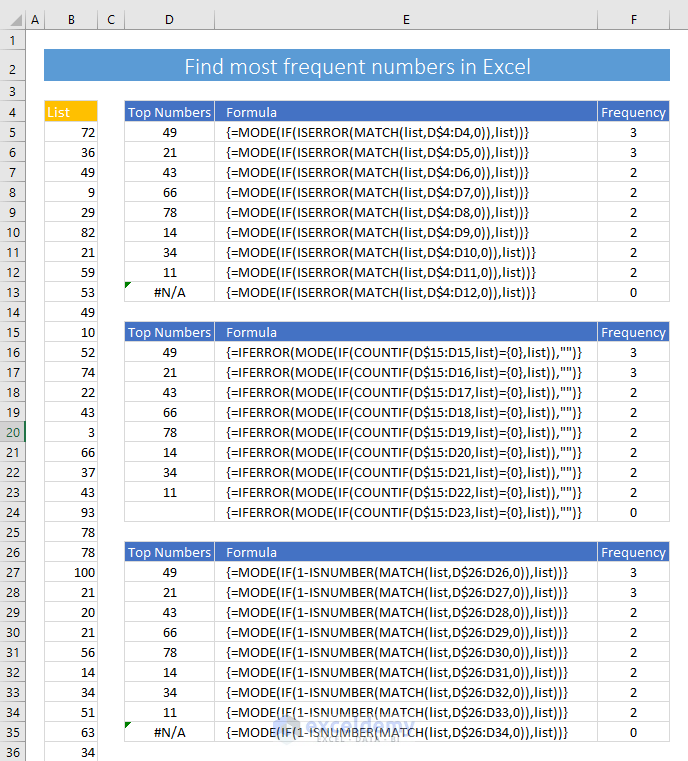In this tutorial, I will show how to find the 5 most (or less or more) frequent numbers in Excel.

I will use Excel formula to do so.

Let’s start.

Suppose, I have a list of 50 numbers and I have created a named range (list) for that list of numbers.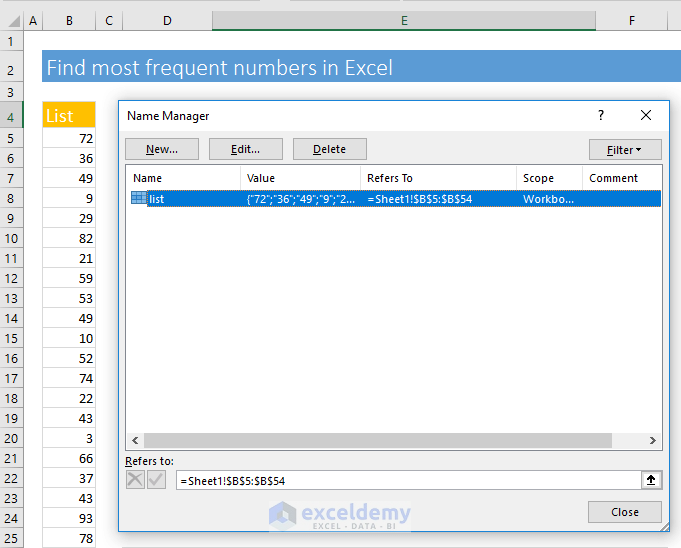We are going to find out the most frequent numbers in the list.

If a number is present more than once in the list, then it will be treated as a frequent number.

## Finding out the most frequent numbers in a list (using Excel formulas)

I will show you 3 Excel formulas that can be used to find out the most frequent numbers in a list.

### Formula 1: Using MODE, IF, ISERROR, and MATCH Functions

To understand this formula, you have to understand how the following Excel functions work:

• MODE
• IF
• ISERROR
• MATCH

Step 1:

In the cell D5, I input this Excel formula:

`=MODE(IF(ISERROR(MATCH(list,D\$4:D4,0)),list))`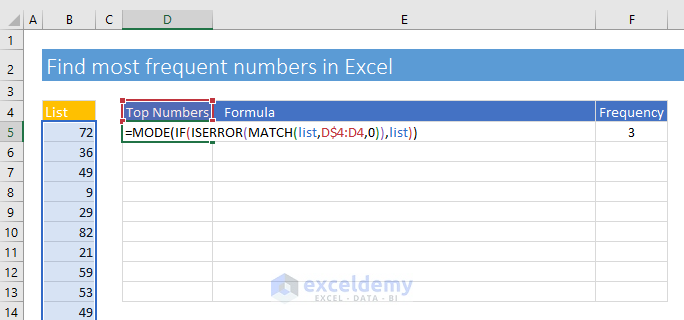Step 2:

I will input this formula as an Excel array formula. So, I press CTRL, SHIFT, and ENTER simultaneously. You see that the formula is Entered as an array formula and the output is 49.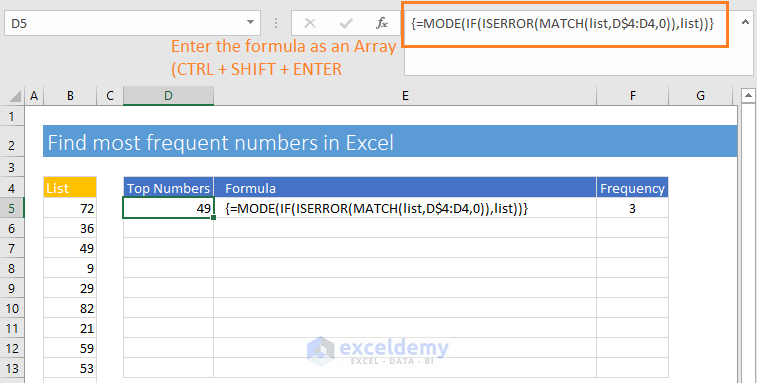Step 3:

Now apply the formula to other cells in the column using the Excel’s Fill Handle Tool.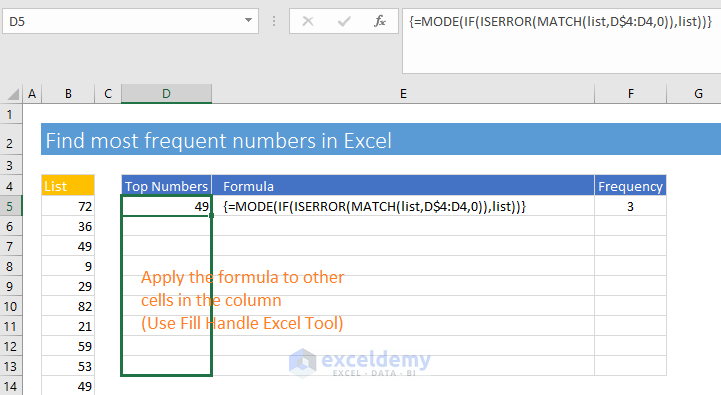You see the outputs. All the numbers that repeated more than once are showing in the column. On the right, you’re seeing the formulas. And on the rightmost column, I have shown the frequency of the number in the list (using Excel COUNTIF function).

At the last cell of the column, you’re seeing an Excel error value (#N/A). It is because Excel’s MODE function does not find a number with frequency more than 1. Don’t worry, I will explain step by step how this Excel formula works within a second.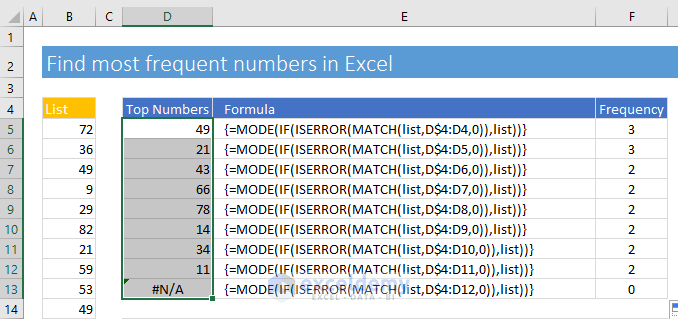How does this formula work?

Let’s explain how this formula actually works.

`=MODE(IF(ISERROR(MATCH(list,D\$4:D4,0)),list))`

Remember, this is an array formula. At first, we shall examine the formula in the cell D5, then the formula in the cell D6.

The formula in the cell D5:

• This is the returned value by the MATCH part: `MATCH(list,D\$4:D4,0)`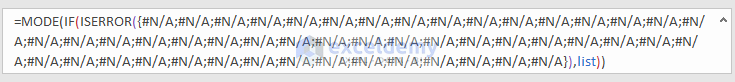This is because in the lookup_array D\$4:D4, MATCH function does not find any match of the list values. So, it returns all the #N/A; errors.

• Then we applied the ISERROR function to these values. ISERROR function returns TRUE if the value is an ERROR.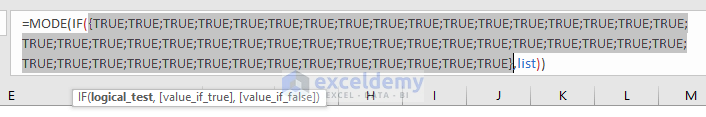• Now the IF Function part. For the first TRUE value, it returns the first number from the list. For the second TRUE value, it returns the second number from the list. And so on. This is the output: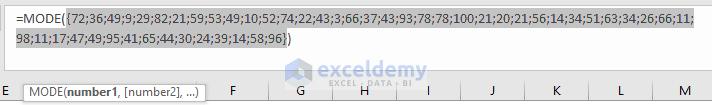• And now the last function MODE. MODE function applies on the list of numbers and returns the most frequent number 49. You see that 21 also has the same frequency, but at first, it returns 49. It is because 49 is the first found in the list.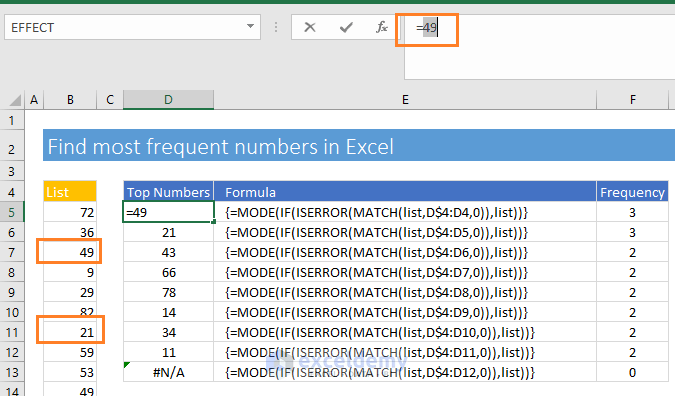The Formula in the cell D6:

`=MODE(IF(ISERROR(MATCH(list,D\$4:D5,0)),list))`

Observe this formula carefully.

You see that the lookup_arry has now been changed to D\$4:D5 as we have extended the formula to this cell from cell D5. So, the lookup_array has changed relatively.

• This time MATCH function returns these values. When the MATCH function finds 49 in the lookup_array (D\$4:D5), it returns the position in the lookup_array. The position of 49 is actually 2.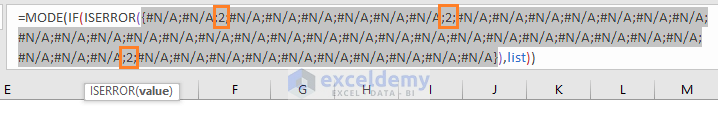• When ISERROR function will get values 2 in the array, it will return FALSE values in those places.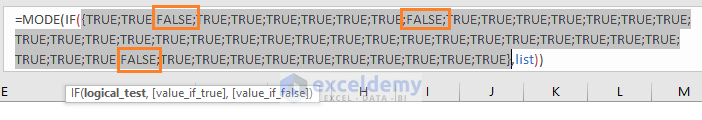• For those FALSE values, IF function will also return the FALSE So, the MODE function will be applied only on the numberical values. Not on the FALSE values.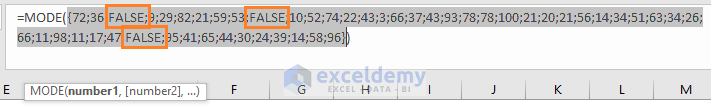• And this is the final output. MODE function returns value 21, the second most frequent numbers in the list.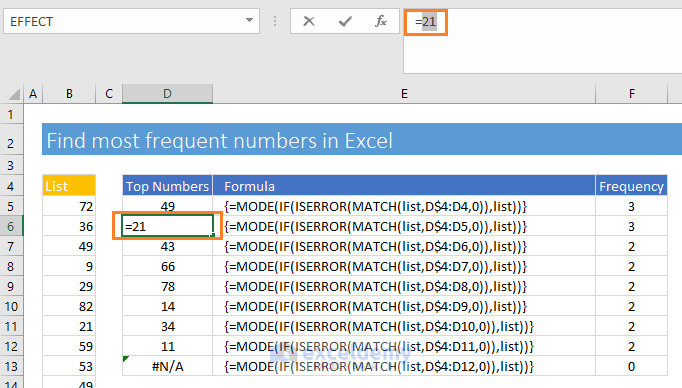I think you can now understand how the formula actually works.

### Formula 2: Using IFERROR, MODE, IF, COUNTIF functions

In this method, we shall use the following Excel functions:

• IFERROR
• MODE
• IF
• COUNTIF

This is the formula I have used in the cell D16:

`=IFERROR(MODE(IF(COUNTIF(D\$15:D15,list)={0},list)),"") `

Entered the formula into the cell as an array formula (press CTRL + SHIFT + ENTER to enter the formula). Then apply the formula to other cells in the column (in the above way).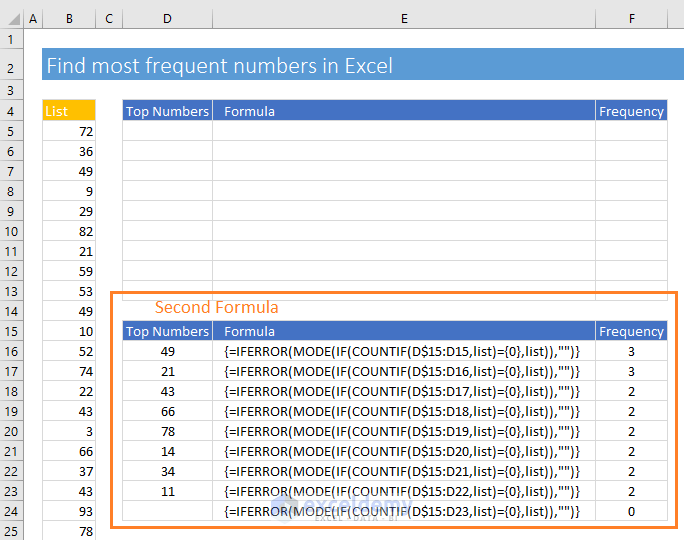I will not explain how this formula works. If you reverse engineer this formula using the above procedure (check out the first formula), then you will be able to understand how this formula works.

### Formula 3: Using MODE, IF, ISNUMBER, MATCH

To build this formula, we have used the following Excel functions:

• MODE
• IF
• ISNUMBER
• MATCH

And this is the formula I have used in the cell D27:

`=MODE(IF(1-ISNUMBER(MATCH(list,D\$26:D26,0)),list)) `

This is also an Excel array formula. So, I have entered this formula pressing the CTRL + SHIFT + ENTER keys together.

Then I have applied this formula to other cells in the column using the Fill Handle Tool. This is the result: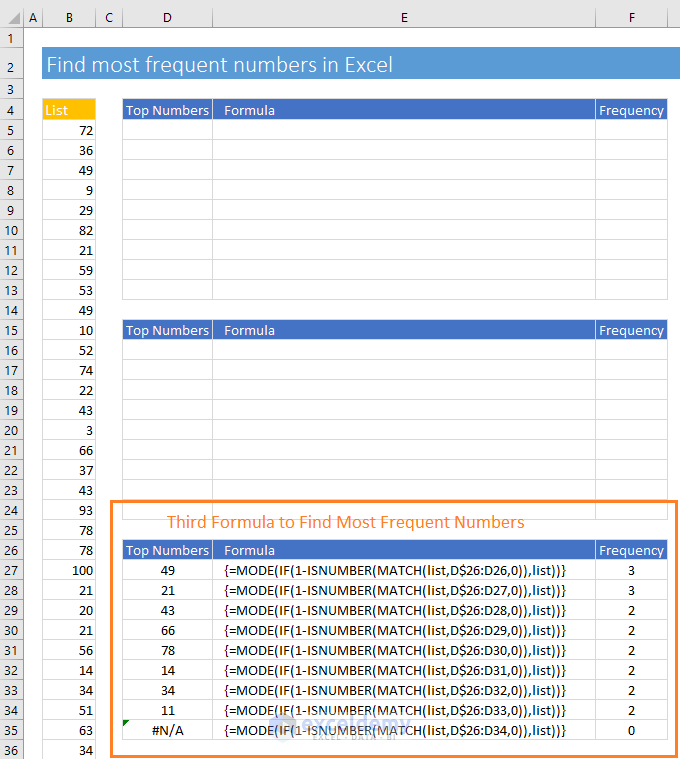I will also not explain how this formula works. If you reverse engineer this formula using the above procedure (check out the first formula), then you will be able to understand how this formula works.

## Conclusion

So, these are my formulas to find the most frequent numbers in a list. I have explained how the first formula works step by step. This is a general process of reverse-engineering an Excel formula. So, I hope you can understand how the other two formulas are working.

Do you know any better way to find 5 of the most frequent numbers in Excel? Let me know in the comment box.

Hello!
Welcome to my Excel blog! It took me some time to be a fan of Excel. But now I am a die-hard fan of MS Excel. I learn new ways of doing things with Excel and share here. Not only how to guide on Excel, but you will get also topics on Finance, Statistics, Data Analysis, and BI. Stay tuned!

1. ReplyFrank May 21, 2019 at 8:10 PM

Thanks a lot this has been a challenge to me for some time now.

Frank

• ReplyMay 21, 2019 at 9:54 PM

You’re welcome, Frank! Feeling good to hear that this article helped you.
Best regards

2. ReplyDennis Paul Eagan Jul 25, 2019 at 5:37 PM

Thank you for this and all your information. I do appreciate it all. Dennis

• ReplyJul 25, 2019 at 6:33 PM

You’re most welcome, Dennis!
Thanks for the nice words.
Best regards
Kawser Ahmed

3. ReplyKKRAO Oct 19, 2019 at 9:07 PM

In the first sight, I did not understand the usage of this formula, Sorry.
Where it is useful, in what contest? If it is finding the numbers, we can sort them in ascending or descending order then we can see the contents of the row.
I request you please enlighten me.

4. ReplyWayne Edmondson Oct 29, 2019 at 11:01 AM

Hi Kawser.. excellent. I was aware of one of your methods.. but learned a lot interrogating the other two. Note for users that you can use MODE() .. the older function or MODE.SNGL() .. the newer function. Either works.. but generally I try and use the newer functions to train my brain to go forward. Thanks for sharing all of your knowledge and skills in the blog posts.. very helpful and appreciated. Thumbs up!